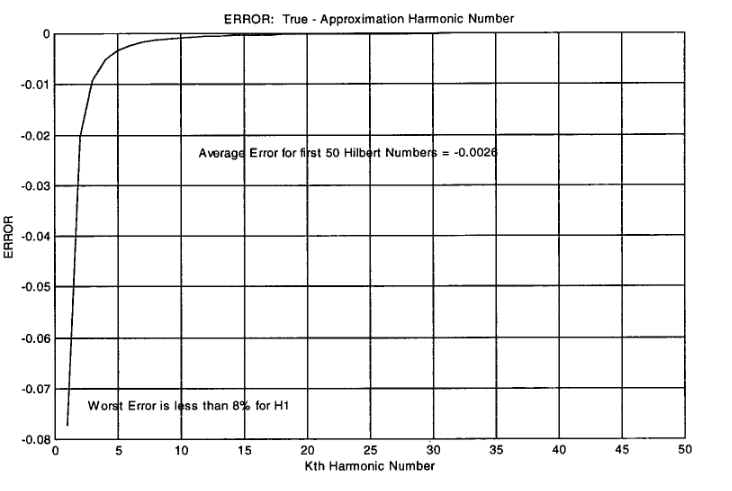Hilbert Matrix Analyses

Ronald Yannone

In my work I use MATLAB (stands for matrix laboratory) for systems engineering design and modeling. MATLAB is a high-performance language for technical computing, developed by MathWorks, Inc. MATLAB integrates computation, visualization, and programniing in an easy-to-use environment where problems and solutions are expressed in familiar mathematical notation. My software library includes MATLAB version 5.1, the Signal Processing toolbox, Statistics toolbox and Symbolic Math toolbox.

I took some time to perform some preliminary analyses of Hilbert matrices using the Symbolic Math toolbox. The first four Hilbert matrices are:

 Hl =  H2 = [1, 1/2] H3 = [1, 1/2, 1/3] H4 = [1, 1/2,1/3,1/4] [1/2, 1/3] [1/2, 1/3, 1/4] [1/2,1/3,1/4,1/5] [1/3, 1/4, 1/5] [1/3,1/4,1/5,1/6] [1/4,1/5,1/6, 1/7]

If we add all the reciprocals of each element in a Hilbert matrix, a nice pattern results. Applying this to the first 10 Hilbert matrices, we get the first ten terms of the sequence:

1, 8, 27, 64, 125, 216, 343, 512, 729, 1000

These are the cubes of the natural numbers. If the cumulative sums of the numbers in this sequence are computed, we get a new sequence, whose first ten terms are:

1, 9, 36, 100, 225, 441, 784, 1296, 2025, 3025

These are the squares of the triangular numbers [numbers of the form (n2+n)/2]. If we add all the squares of the reciprocals of each element in a Hilbert matrix, we get the first ten terms of the sequence:

1, 18, 93, 296, 725, 1506, 2793, 4768, 7641, 11650

Taking successive differences of this sequence four times results in constant differences of 28. The resulting 4h-order polynomial expression is:

 1 _ [7N4 - N2] 6

If we take the reciprocals of the elements of the Hilbert matrix, square the resulting matrix, and compute the sum of all the resulting elements in the product, we get the first ten terms of the sequence:

1, 34, 261, 1104, 3375, 8406, 18179, 35456, 63909, 108250

Taking successive differences of this sequence five times results in constant differences of 130. The resulting 5h-order polynomial expression is:

 1 _ [13N5 - N3] 12

If we take the combined sum of the main and anti-main diagonals of the reciprocal Hilbert matrix elements, we get the following first ten terms of the sequence:

2, 8, 18, 32, 50, 72, 98, 128, 162, 200

Taking successive differences twice results in constant differences of 4. The resulting 2nd-order polynomial expression is:

2N2

Returning to the first four Hilbert matrices, we can see an interesting feature in the structure of the rows (or columns) of the Nth order Hilbert matrix. Namely, we see that the first row (or column) are the Nth harmonic numbers. The first four harmonic numbers are:

H1 = 1, H2 = 1 + 1/2, H3 =1 + 1/2 + 1/3, H4 = 1 + 1/2 + 1/3 +1/4

In general, the nth harmonic number is

Hn = 1 + 1/2 + 1/3 + . . . + 1/n

No harmonic number is a whole number after the first one: look at the term with the highest power of 2 in it. It has nothing with which to pair. So, H2, H3, H4, . . . have odd numerator and even denominator. A closed form approximation for the nth harmonic number is given by

Hn = ln(n) + gamma + 1/2n,

where gamma = 0.577215664901532860606512, the Euler-Mascheroni numher.

The first 50 true and approximate harmonic numbers are plotted in Figure 1 and the error (difference) between them is shown in Figure 2.Figure 1. The First 50 True and Approximate Harmonic NumbersFigure 2. Average Difference (Error) for the First 50 True and Approximate Harmonic Numbers

The successive rows (or columns) in the Hilbert matrices are larger harmonic numbersthe summation of the first so many harmonic numbers. E.g., in the second Hilbert matrix, the first row houses the second harmonic number: 1 + 1/2 = 3/2. The second row houses the third harmonic number minus the first (i.e., no 1 term), or 1.8333 (3rd harmonic number) - 1 = 0.8333which is 1/2 + 1/3.

The sum of all elements in the first 20 Hilbert matrices are:

 1 2.3333 3.7000 5.0762 6.4563 7.8385 9.2219 10.6059 11.9905 13.3754 14.7606 16.1459 17.5314 18.9170 20.3027 21.6885 23.0744 24.4602 25.8462 27.2321

There are probably many more interesting properties of Hilbert matrices. If you know of any, please share them with us. Thanks, Ron Yannone

See the MATLAB code for the analysis reported in this article, item 15 in this issue.